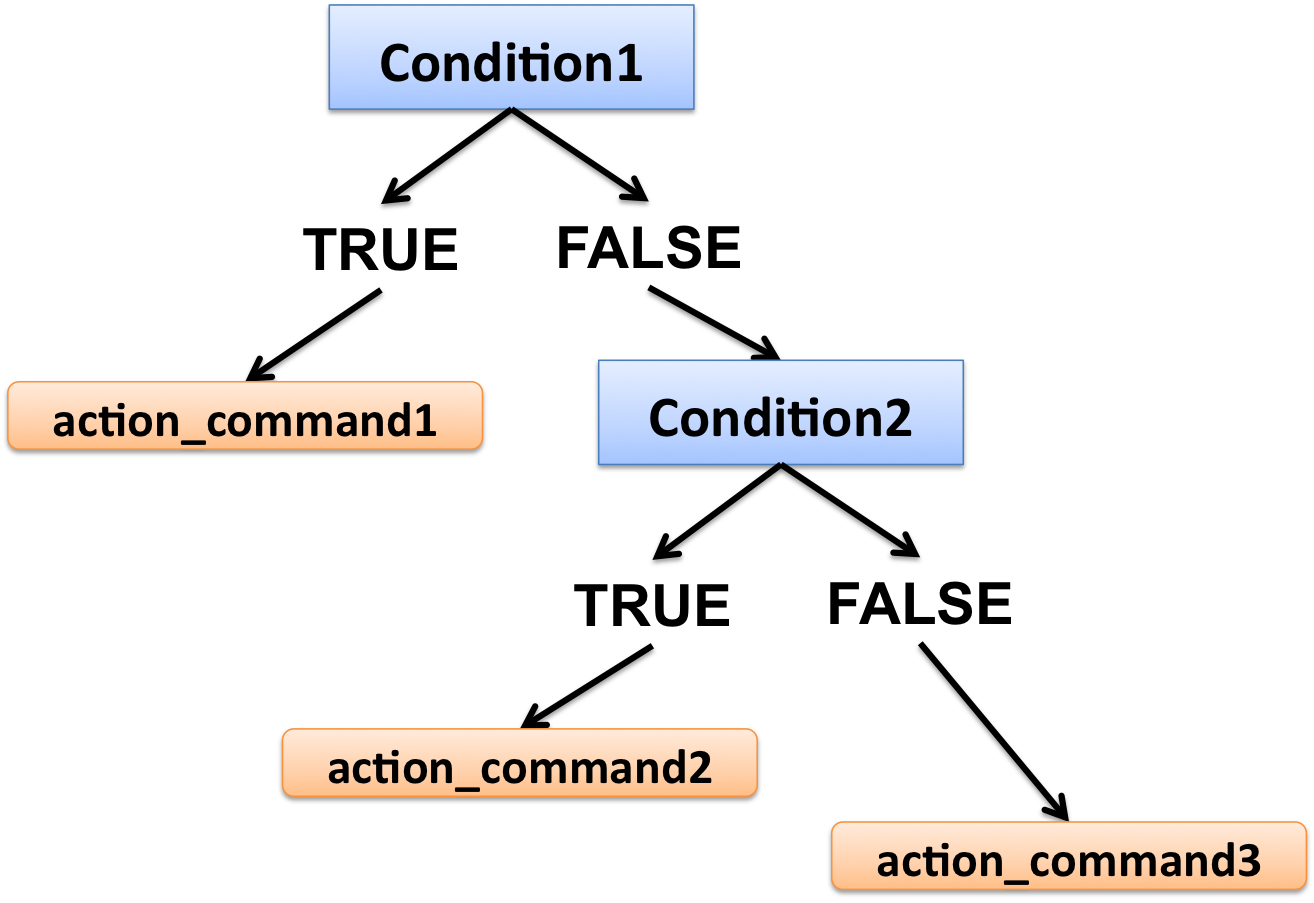# Part14 Conditional statement

Conditional statements are expressions that perform different computations or actions depending on whether a predefined boolean condition is TRUE or FALSE.

### “if” statement

Structure of the if statement:

``````if(condition){
action_command
}``````

If the condition is TRUE, then proceed to the action_command; if it is FALSE, then nothing happens.
Note the usage of curly brakets {} to start and end the conditional!

``````k <- 10

# print if value is > 3
if(k > 3){
print(k)
}

# print if value is < 3
if(k < 3){
print(k)
}``````

#### With else

``````if(condition){
action_command1
}else{
action_command2
}``````

If the condition is TRUE, then proceed to action_command1; if the condition is FALSE, proceed to action_command2.

``````k <- 3

if(k > 3){
print("greater than 3")
}else{
print("less than 3")
}``````

#### With else if

``````if(condition1){
action_command1
}else if(condition2){
action_command2
}else{
action_command3
}``````If the condition1 is TRUE, then proceed to the action_command1; if the condition1 is FALSE, test for condition2: if the condition2 is TRUE, proceed to the action_command2; if neither condition1 nor condition2 are TRUE, then proceed to the action_command3 (else).

Note that you can add up as many else if statements as you want, but only one else (not compulsory either).

• Example without else
``````k <- -2
# Test whether k is positive or negative or equal to 0
if(k < 0){
print("negative")
}else if(k > 0){
print("positive")
}else if(k == 0){
print("is 0")
}``````
• Example with else
``````k <- 10

# print if value is <= 3
if(k <= 3){
print("less than or equal to 3")
}else if(k >= 8){
print("greater than or equal to 8")
}else{
print("greater than 3 and less than 8")
}``````